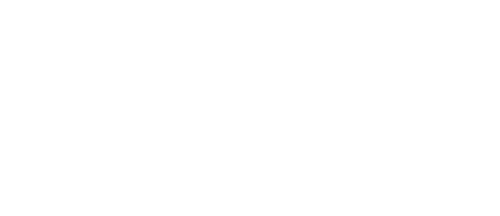Apply Now!### MATH135M - Numerical Algebra and Trigonometry (3-0-3)

Provides students with the basic algebra and trigonometry manipulatives to compute solutions in their curricula. Algebra topics offered are signed numbers, polynomial operations, solutions of linear equations involving numerical and literal terms, word problems and formula manipulation. Trigonometric topics and trigonometric ratios as applied to right triangles and computation of measures in oblique triangles, using the Law of Sines and the Law of Cosines. Prerequisite: MATH111M with a grade of “C” or better or permission of the instructor. Offered every semester.